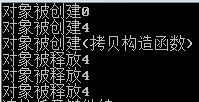# C++ 类(构造函数和析构函数)

## 文章概述

1. 构造函数和析构函数的由来？
2. 构造函数和析构函数的基本语法
3. C++编译器构造析构方案 PK 对象显示初始化方案
4. 构造函数的分类以及调用
5. 默认的构造函数
6. 构造函数调用规则
7. 构造函数和析构函数的总结

## 构造函数和析构函数的基本语法

a. 构造函数:

• C++中的类需要定义与类名相同的特殊成员函数时,这种与类名相同的成员函数叫做构造函数;
• 构造函数可以在定义的时候有参数;
• 构造函数没有任何返回类型。
• 构造函数的调用: 一般情况下,C++编译器会自动的调用构造函数。特殊情况下,需要手工的调用构造函数。
class Test
{
public:
//构造函数
Test()
{

}
}

b. 析构函数:

• C++中的类可以定义一个特殊的成员函数清理对象,这个特殊的函数是析构函数;
• 析构函数没有参数和没有任何返回类型;
• 析构函数在对象销毁的时候自动调用;
• 析构函数调用机制: C++编译器自动调用。
class Test
{
~Test()
{

}
}

## C++编译器构造析构方案 PK 对象显示初始化方案

class Test
{
private:
int x;
public:
Test(int x)
{
this->x = x;
cout << "对象被创建" << endl;
}
Test()
{
x = 0;
cout << "对象被创建" << endl;
}
void init(int x)
{
this->x = x;
cout << "对象被创建" << endl;
}
~Test()
{
cout << "对象被释放" << endl;
}
int GetX()
{
return x;
}
};

int main()
{
//1.我们按照C++编译器提供的初始化对象和显示的初始化对象
Test a(10);
Test b;      //显示创建对象但是还是调用无参构造函数,之后显示调用了初始化函数
b.init(10);

//创建对象数组（使用构造函数）
Test arr = {Test(10),Test(),Test()};
//创建对象数组 (使用显示的初始化函数)
Test brr;   //创建了3个对象,默认值
cout<<brr.GetX()<<endl;
brr.init(10);
cout<<brr.GetX()<<endl;
return 0;
}

• a. init函数只是一个普通的函数,必须显示的调用;
• b. 一旦由于失误的原因,对象没有初始化,那么结果也是不确定的。没有初始化的对象,其内部成员变量的值是不定的;
• c. 不能完全解决问题。

## 构造函数的分类以及调用

a. 无参构造函数

class Test
{
private:
int x;
public:
Test()
{
this->x=10;
}
}

b. 有参构造函数

class Test
{
private:
int x;
public:
Test(int x)
{
this->x=x;
}
}

• Test a(10); 调用有参数构造函数
• Test b=(2,3); 逗号表达式的值是最后一位,调用有参数构造函数
• Test c=Test(2); 产生一个匿名对象,直接转化成c（只会调用一次有参数构造函数）

c. 拷贝构造函数: 使用对象a初始化对象b

class Test
{
private:
int x;
public:
Test(const Test& a)
{
this->x=a.x;
}
}

class Test
{
private:
int x;
public:
Test(int x)
{
this->x = x;
cout << "对象被创建" << endl;
}
Test()
{
x = 0;
cout << "对象被创建" << endl;
}
~Test()
{
cout << "对象被释放" << endl;
}
Test(const Test& a)
{
this->x = a.x;
cout << "对象被释放（拷贝构造函数）" << endl;
}
};
• 第一个场景: 用对象a初始化对象b:
Test a(10);
//调用的是拷贝构造函数
Test b = a;
• 第二个场景: 用对象a初始化对象b
Test a(10);
//调用的是拷贝构造函数
Test b(a)

• 第三个场景: 实参初始化形参的时候,会调用拷贝构造函数
    Test a(10);
//实参初始化形参
PrintF(a);
• 第四个场景: 函数返回一个匿名对象
我们分析第四个场景前,我们看看下面的这个全局函数:
//该函数返回的是谁?
Test p()
{
Test c(4);
return c;
}a. 匿名对象初始化另一个对象:

Test a=p();b. 匿名对象赋值时:

    Test b;
//匿名对象赋值另一个对象
b = p();a. 匿名对象初始化另一个对象时,匿名对象直接变成有名的对象(初始化的那个对象);
b. 匿名对象赋值另一个对象时,匿名对象赋值成功会被析构。

## 默认的构造函数

a. 默认的无参数构造函数: 当类中没有定义构造函数时,编译器会提供一个无参数构造函数,并且函数体为空;
b. 默认的拷贝构造函数: 当类中没有定义拷贝构造函数时,编译器会提供一个默认的拷贝构造函数,简单的进行成员变量的值复制。

## 构造函数调用规则

a. 当类中没有定义一个构造函数的时候,C++编译器会提供默认的无参数构造函数和拷贝构造函数;
b. 当类中定义了拷贝构造函数,C++编译器不会提供无参数构造函数;
c. 当类中定义了任意的非拷贝构造函数,C++编译器不会提供默认的无参数构造函数;
d. 默认的拷贝构造函数只是进行成员变量的简单赋值;

## 构造函数和析构函数的总结

a. 构造函数时C++中用于初始化对象状态的特殊函数;
b. 构造函数在对象创建的时候自动调用;
c. 构造函数和普通成员函数都遵循重载原则;
d. 拷贝构造函数是对象正确初始化的重要保障;
e. 必要的时候必须手工的写拷贝构造函数。

-

©️2019 CSDN 皮肤主题: 书香水墨 设计师: CSDN官方博客